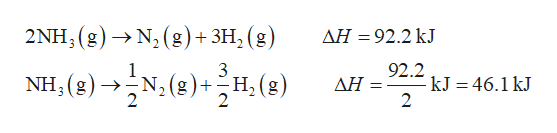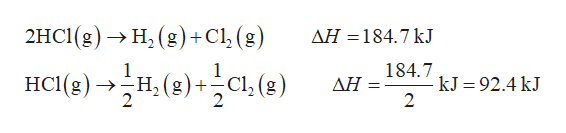# N2(g) + 3H2(g) → 2NH3(g)ΔH = -92.2 kJH2(g) + Cl2(g) → 2HCl(g)ΔH = -184.7 kJN2(g) + 4H2(g) + Cl2(g) → 2NH4Cl(s)ΔH = -628.9 kJFind the ΔH of the following reaction:NH3(g) + HCl(g) → NH4Cl(s)

Question

N2(g) + 3H2(g) → 2NH3(g)ΔH = -92.2 kJ

H2(g) + Cl2(g) → 2HCl(g)ΔH = -184.7 kJ

N2(g) + 4H2(g) + Cl2(g) → 2NH4Cl(s)ΔH = -628.9 kJ

Find the ΔH of the following reaction:

 NH3(g) + HCl(g) → NH4Cl(s)
check_circle

Step 1

Hess’s law states that the total enthalpy change of a reaction remains same whether the reaction occurs in one step or in a number of steps.

According to Hess’s law,

1. The enthalpy change of a reaction becomes half, if its coefficients are divided by 2.
2. The sign of enthalpy change gets reversed on reversing the chemical reaction.
3. The enthalpy change of a reaction becomes twice, if its coefficients are multiplied with 2.
Step 2

Reverse the first reaction and divide it by 2.help_outlineImage Transcriptionclose2ΝΗ, ( g) >N, (g)+3H, (g AH 92.2 kJ 92.2 -kJ = 46.1 kJ 2 NH (g)N2(g)+H(g) ΔΗ- fullscreen
Step 3

Reverse the second reaction...help_outlineImage Transcriptionclose2HC(g) -> Н, (g)+Cl1,(g) ΔΗ-184.7 kJ нсце) -н.е)с, е) 184.7 -Н, (g) +—Cl, (g) kJ 92.4 kJ 2 ΔΗ fullscreen

### Want to see the full answer?

See Solution

#### Want to see this answer and more?

Solutions are written by subject experts who are available 24/7. Questions are typically answered within 1 hour.*

See Solution
*Response times may vary by subject and question.
Tagged in

### Chemistry